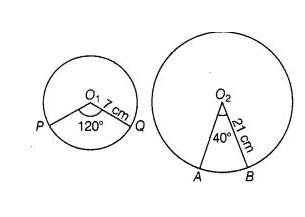# The central angles of two sectors of circles

Question:

The central angles of two sectors of circles of radii 7 cm and 21 cm are respectively 120° and 40°. Find the areas of the two sectors as well as the

lengths of the corresponding arcs. What do you observe?

Solution:

Let the lengths of the corresponding arc be l1 and l2Given that, radius of sector $P O, O P=7 \mathrm{~cm}$

and radius of sector $\mathrm{AO}_{2} \mathrm{BA}=21 \mathrm{~cm}$

Central angle of the sector $P O, Q P=120^{\circ}$

and central angle of the sector $\mathrm{AO}_{2} \mathrm{BA}=40^{\circ}$

$\therefore$ Area of the sector with central angle $\mathrm{O}_{1}$

$=\frac{\pi r^{2}}{360^{\circ}} \times \theta=\frac{\pi(7)^{2}}{360^{\circ}} \times 120^{\circ}$

$=\frac{22}{7} \times \frac{7 \times 7}{360^{\circ}} \times 120$

$=\frac{22 \times 7}{3}=\frac{154}{3} \mathrm{~cm}^{2}$

and area of the sector with central angie $\mathrm{O}_{2}$

$=\frac{\pi r^{\circ}}{360^{\circ}} \times \theta=\frac{\pi(21)^{2}}{360^{\circ}} \times 40^{\circ}$

$=\frac{22}{7} \times \frac{21 \times 21}{360^{\circ}} \times 40^{\circ}$

$=\frac{22 \times 3 \times 21}{9}=22 \times 7=154 \mathrm{~cm}^{2}$

Now, corresponding arc length of the sector $P O, Q P$

$=$ Central angle $\times$ Radius of the sector

$=120^{\circ} \times 7 \times \frac{\pi}{180^{\circ}}$ $\left[\because \theta=\frac{l}{r}\right.$ and $\left.1^{\circ}=\frac{\pi}{180^{\circ}} R\right]$

$=\frac{2}{3} \times 7 \times \frac{22}{7}$

$=\frac{44}{3} \mathrm{~cm}$

and corresponding arc length of the sector $\mathrm{AO}_{2} \mathrm{BA}$

$=$ Central angle $\times$ Radius of the sector

$=40^{\circ} \times 21 \times \frac{\pi}{180^{\circ}} \quad\left[\therefore \theta=\frac{l}{r}\right.$ and $\left.1^{\circ}=\frac{\pi}{180^{\circ}} R\right]$

$=\frac{2}{9} \times 21 \times \frac{22}{7}$

$=\frac{2}{3} \times 22=\frac{44}{3} \mathrm{~cm}$

Hence, we observe that arc lengths of two sectors of two different circles may be equal but their area need not be equal.